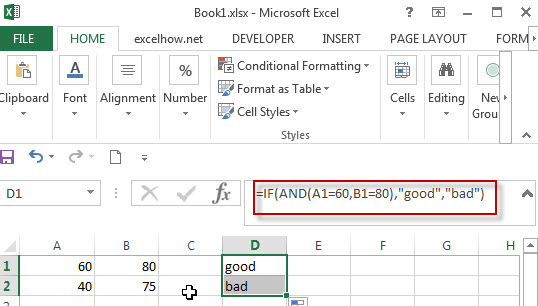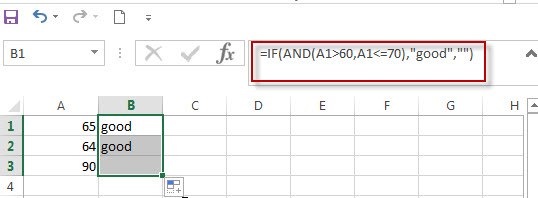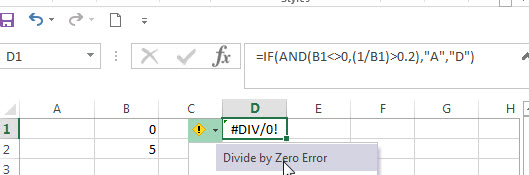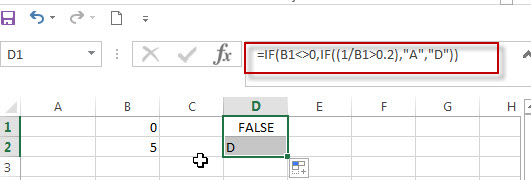# Excel IF formula with AND logical function

This post will guide you how to use IF function combining with AND logical function in Excel.

If you want to check if a value in cell match two or more conditions at the same time, if the test is TRUE, then you can take certain action. You can use the IF function combining with AND function to construct a condition statement. If the test is FALSE, then take another action.

## Excel formula using IF & AND function

The syntax of AND function in excel is as follow:

`=AND(condition1,[condition2],...)`

The Excel AND function returns TRUE if all of arguments are TRUE, and it returns FALSE if any of arguments are FALSE.

Now we want to check the results of two exam scores where the first score in cell A1 is greater than or equal to 60 AND the second score in cell B1 is greater than or equal to 80. If both conditions are TRUE, then returns the text of “good”, and if FALSE returns “bad”. Based on the above logic, we can write down the below IF formula:

`=IF(AND(A1=60,B1=80),"good","bad")`

We can enter the above formula into cell D1, and then drag the Fill Handle to the range D1:D3.it will apply for the other cells for this formula.The logic test will check the two different cells in the above IF formula, if we can run two or more tests on the same cell. Of course yes, for example, if you want to check cell A1 if the value in cell A1 is greater than 60 but less than or equal to 70. If the test is TRUE, then take one action.

The logical test we can use for above logic as below:

`=AND(A1>60, A1<=70)`

IF formula:

`=IF(AND(A1>60,A1<=70),”good”,””)`In this formula, if the test is FALSE, we just add an empty string (“”), IF the test is TRUE, the test string of “good” will appear.

Note: The AND function will check all test conditions in excel, even if one of the already tested conditions has a value of FALSE. This behavior is different with other programming languages. If any previous test conditions return FALSE, the subsequent conditions will not be tested.

For example: If the value of Cell B1 is 0, then the below IF&AND formula will return error message as “Divide by Zero Error”.

`=IF(AND(B1<>0,(1/B1)>0.2),”A”,”D”))`As mentioned above, the AND function will check all conditions. To avoid this issue, we can use a nested IF function and check if the value of Cell B1 is not equal to 0 in the first IF statement.

The nested if formula is as below:

`=IF(B1<>0,IF((1/B1>0.2),”A”,”D”))`### Related Formulas

• Excel IF formula with equal to logical operator
The “Equal to” logical operator can be used to compare the below data types, such as: text string, numbers, dates, Booleans.  This section will guide you how to use “equal to” logical operator in excel IF formula with text string value and dates value…
• Excel IF formula with OR logical function
If you want to check if one of several conditions is met in your excel workbook, if the test is TRUE, then you can take certain action. You can use the IF function combining with OR function to construct a condition statement…
• Excel IF formula with AND & OR logical functions
If you want to test the result of cells based on several sets of multiple test conditions, you can use the IF function with the AND and OR functions at a time…

### Related Functions

• Excel AND function
The Excel AND function returns TRUE if all of arguments are TRUE, and it returns FALSE if any of arguments are FALSE.The syntax of the AND function is as below:= AND (condition1,[condition2],…) …
• Excel IF function
The Excel IF function perform a logical test to return one value if the condition is TRUE and return another value if the condition is FALSE….
• Excel nested if function
The nested IF function is formed by multiple if statements within one Excel if function. This excel nested if statement makes it possible for a single formula to take multiple actions…